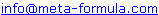# Metastock Formulas - N Click here to go back to Metastock Formula Index

 IMPORTANT: These formulas aren't my complete collection. For my complete collection of instantly usable, profitable and powerful MetaStock formulas Click Here

Would You Like To Use MetaStock To Its Full Potential? Discover the Amazingly Simple Secret to Master Metastock Step-By-Step - click here

A | A 1 | B | B 1 | C | C 1 | D | D 1 | E | F | G | H | I | J | K | L | M | M 1 | N | O | P | R | S | S 1 | T | U | V | W | Z |

 Natenberg's Volatility rev. 01/21/97 Historical volatility is defined by Sheldon Natenberg, as the standard deviation of the logarithmic price changes measured at regular intervals of time. In Mr. Natenberg's book, "Option Volatility & Pricing," he covers volatility in detail and gives the formula for computing historical volatility. In MetaStock, the equivalent formula would be: Std( Log( C / Ref( C ,-1 ) ) ,10 ) * Sqrt( 365 / 7 ) The above assumes Weekly Data. To utilise this with Daily Data, the MetaStock formula would be: Std( Log( C / Ref( C,-1) ),10 ) * Sqrt( 365 ) For further interpretation refer to the book "Option Volatility & Pricing," by Sheldon Natenberg. Nat's Volt Std(log(c/ref(c,-1)),10)*sqr(365/7) (Go Top) NR4 Formula from Trading Tactics page 100 Column A Std(Log(C/Ref(C,-1)),5)/Std(Log(C/Ref(C,-1)),99) Column B HIGH-LOWRef(LOW,-1)) Column D HIGH Column E LOW Filter colA<.5 AND (colB= 1 OR colC= 1) (Go Top)

If you have Metastock formulas you would like to share,
Please email toWe look forward to hearing from you!